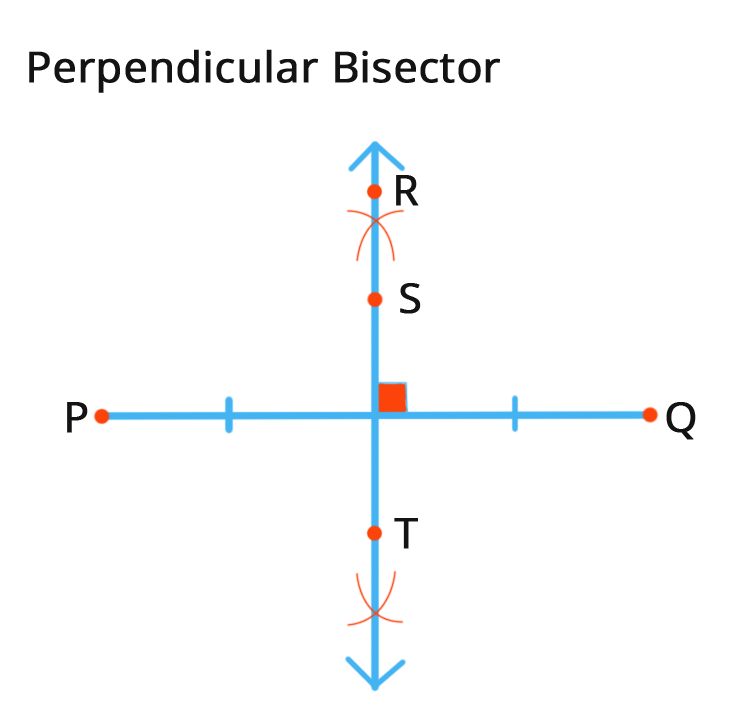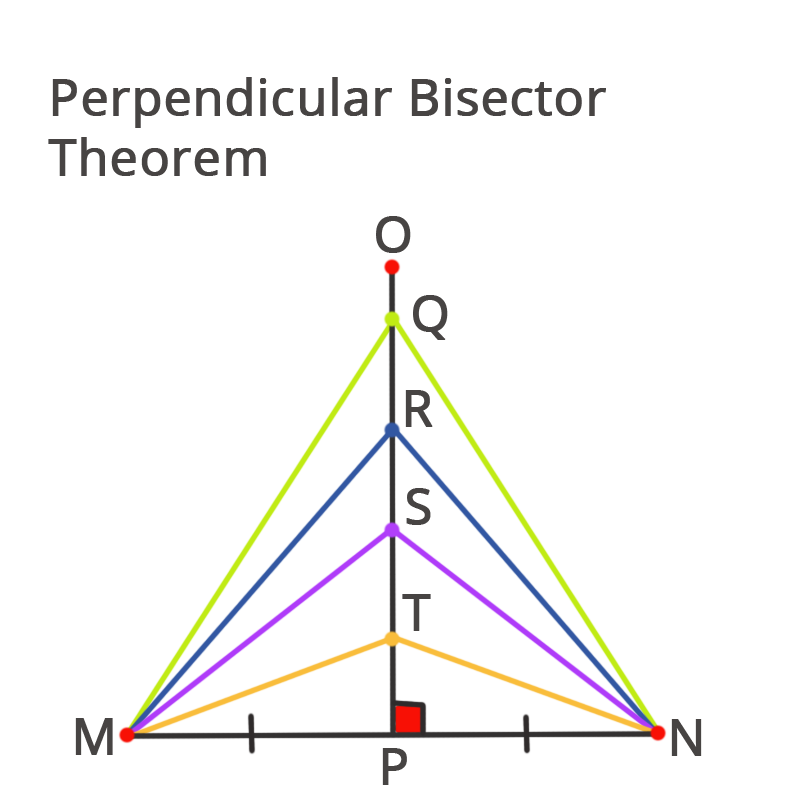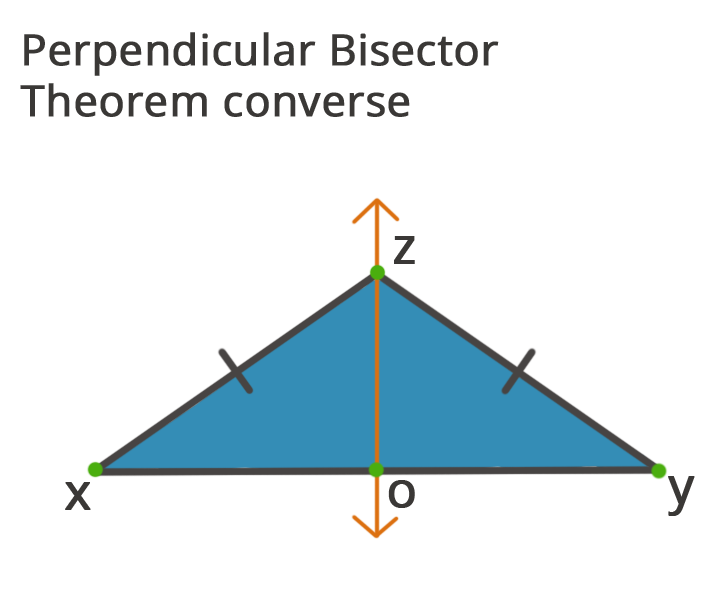• Call Now

1800-102-2727•

# Perpendicular Bisector Theorem

The perpendicular of a line segment is defined as a line that splits another line segment into two halves via its midpoint at
90o. Any point on the perpendicular bisector is equidistant from both endpoints of the line segment on which it is drawn, according to the perpendicular bisector theorem. If a pillar stands at an angle in the middle of a bridge, all of the pillar's points will be equidistant from the bridge's endpoints.

The perpendicular bisector is a line segment that splits a line into equal size pieces by intersecting it perpendicularly. When two lines cross in such a way that they create a 90-degree angle with one another, they are called as being perpendicular to each other. A bisector, on the other hand, splits a line into two equal halves. A perpendicular bisector of a line segment AB crosses AB at 90 degrees thus divides it into two equal pieces.

## What is a Perpendicular Bisector, and how does it work?

A perpendicular bisector is a line segment that meets another line segment at a straight angle and splits it in half at its midway.

• It bisects or splits AB into two equal parts.
• It is perpendicular to (or makes right angles with) AB.
• Inside the perpendicular bisector, each point is equal to point A and B.

When dealing with practical geometry, you'll frequently come across the use of perpendicular bisectors, such as when drawing an isosceles triangular or determining the center of a circle. The methods for making a perpendicular bisector of a line with a compass as well as a ruler are outlined below.## What is the Perpendicular Bisector Theorem, and how does it work?

Any point on the perpendicular bisector is equidistant from both endpoints of the line segment on which it is drawn, according to the perpendicular bisector theorem.## What is the Perpendicular Bisector Theorem's inverse?

The inverse of the perpendicular bisector theorem says that a point is on the perpendicular bisector of a line segment if it is equidistant from both endpoints of the line segment in the same plane.

## Important Reminders

• The SAS congruence criteria may be used to prove the perpendicular bisector theorem and its converse.
• The perpendicular bisector theorem is utilized in the construction of various architectural entities as well as in designing structures that must be built in the center and at equal distances from both ends.Perpendicular Bisector Theorem is extensively discussed in NCERT Solutions Class 9 Maths Chapter 11 Constructions along with geometric tools, their characteristics, and usage.

What Is a Perpendicular Bisector and what does it mean?
A perpendicular bisector is a line segment that cuts another line at a 90-degree angle. A perpendicular bisector, in other words, crosses another line at 90 degrees and splits it into equal size halves.

Is it possible for a Perpendicular Bisector to be the Median of a Triangle?
A perpendicular bisector can, in fact, be a triangle's midpoint. A median is a line segment that runs from a triangle's vertex to the middle of the side opposite that vertex. A perpendicular bisector of half is formed when the median intersects the opposing side at 90 degrees. For example, the medians of a triangle have always been perpendicular bisectors.What is the name of the position where a triangle's perpendicular bisectors meet?
A circumcenter of a triangular is the place where the perpendicular bisectors of a triangular intersect, and it is halfway from all the points.

What is called the Perpendicular Bisector Theorem, and how does it work?
According to the perpendicular bisector theorem, if such a point is upon a section's perpendicular bisector, it is equal from the section's ends.

Talk to our expert
Resend OTP Timer =
By submitting up, I agree to receive all the Whatsapp communication on my registered number and Aakash terms and conditions and privacy policy